Clutch Prep is now a part of Pearson
Ch. 1 - A Review of General ChemistryWorksheetSee all chapters

# Lewis Structure

See all sections
Sections
Intro to Organic Chemistry
Atomic Structure
Wave Function
Molecular Orbitals
Sigma and Pi Bonds
Octet Rule
Bonding Preferences
Formal Charges
Skeletal Structure
Lewis Structure
Condensed Structural Formula
Degrees of Unsaturation
Constitutional Isomers
Resonance Structures
Hybridization
Molecular Geometry
Electronegativity
Isomer
Polar Vs. Nonpolar
Covalent Bond
Polar Bond

Drawing Lewis Structures is something we learned how to do in Gen Chem. Nothing has changed since then, but now since we know about bonding preferences, that makes it a lot easier.

###### Lewis Structure Rules

Concept #1: How to use Organic Chemistry to make Lewis Structures easier.

Transcript

Remember that Lewis structures were ways to describe molecules that everything is drawn explicitly so that means all the bonds, all the lone pairs, all the atoms were drawn out perfectly explicitly so you can see everything on the structure.
Back in the day, you learned a set of rules in gen chem that you were supposed to remember about computing theoretical number of valence electrons then subtracting electrons and stuff like that, but if you already have a knowledge of the octet rule and if you already have knowledge of bonding preferences, it turns out that we can do almost all these Lewis structures just on that knowledge.
So I'm going to go through these rules just for the sake of reminding you, but I'm also going to show you that now in organic chemistry we have an easier method to do this based on the new information that you've learned about bonding preferences.
I'm just going to go over these rules really fast. Remember that the first thing we always had to do was if you're given a molecular formula and you're trying to convert that into a Lewis structure and trying to figure out what does the Lewis structure look like, you always start with the atom that has the highest bonding preference in the middle.
For those of you maybe in your class you may have learned it somewhat different. You may have learned that you start with the most electropositive atom. That's fine. Both of them work. I just want to make sure that you always start off with – electropositive just means that the one that is furthest away from fluorine.
In that case, you would just start off with that one or you can start off with the highest bonding preference meaning the one that makes the most bonds. For example, if I were to compare nitrogen versus oxygen, which won would win? Nitrogen because nitrogen likes to have three bonds and oxygen likes to have two. Does that make sense?
So you start off with the highest bonding preference in the middle and then you propose what's called a sigma bond framework. Now I want you guys to pay attention to this word 'propose.' Propose means that this is going to take some trial and error. You're not going to know it usually on the very first try.
What I suggest is don't stress out about this. Just go ahead and write maybe the stupidest thing you can think of at the beginning, just the one that's like maybe the most obvious that that's wrong and then figure out how to fix it. That's the important thing. Hopefully, as we get better, in less and less tries, we're able to get the right structure.
Now, just one more point. If it turns out two atoms have the same bonding preference, so for example, sulfur, I mean, yeah, sure, sulfur and oxygen. They're both in the same group, so sulfur and oxygen like to both have two bonds. Well, then you would just place the bigger one in the center, so that means sulfur would beat oxygen.
Then what you do is you complete all the octets using lone pairs. That means that once you draw the sigma bond framework, you're going to fill everything in with just lone pairs everywhere to complete everyone's octet. So those thing is going to be loaded with dots. It's going to look like it got hit with a shotgun.
Then what we do is there's this complicated, not complicated, but just this math you have to do where you have to calculate the theoretical valence electrons then calculate the actual number of electrons that you see on the page. Then you would subtract the two numbers and get what's called the electron difference. And then if you had a positive electron difference, then you would create double bonds, meaning that if you have too many dots on the page, you start making double bonds to get rid of dots. If you had too few dots, then you start adding more lone pairs in order to satisfy the actual theoretical valence.
That's the rules, but what I want to tell you is that steps three through five, now that we know orgo and now that we know about bonding preferences and formal charges, you can actually just use formal charges and bonding preferences to figure all that out. We're not going to keep doing this math anymore.

*You might have learned a slightly different version, but this is close enough, you get the point.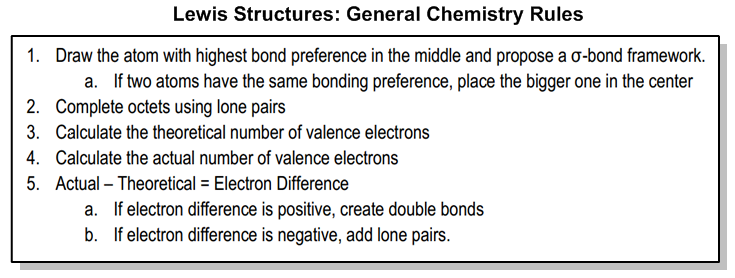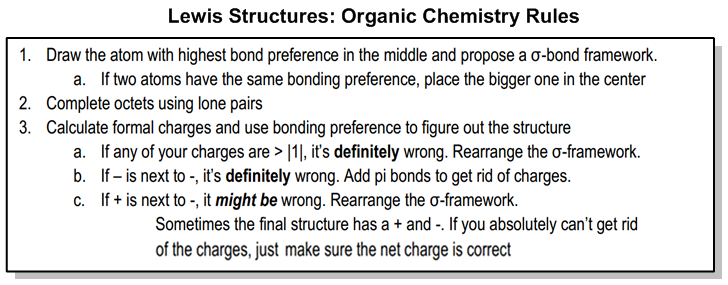Example #1: Drawing the Lewis Structure for N2H4.

Transcript

An example would be a question that says, find the Lewis structure for the compound N2H4. I don't know what that looks like, so let's just go ahead and jump into it.
The first thing I do is I take the four atoms and I say which one has the highest bonding preference. Obviously one of the nitrogens.
What I do is take the one with the highest bonding preference and I put it in the middle and then I propose a sigma bond framework. Notice I even put random here because I'm trying to emphasize that you're not supposed to just know this from the first shot. A lot of students feel confused because they're like, “How am I supposed to know this?” This is trial and error.
What I did here was I literally just made like the dumbest version that I could. I just put a bunch of atoms around the N. So I said, okay, one of the H's can go to this N, the other H can go here, the other H can go here and then one of the N's can go there.
What I'm basically doing is I'm just trying to get the first atom in the middle to fill its octet and then any other atoms, I'm attaching them somewhere else. So basically, I attached four atoms to the N because that's the most that it can handle. I have one hydrogen left over, so then I was like, “Hey, I'll just put it on that N and see what happens.”
Now, if I go to my second rule it says fill in the octets with lone pairs. That first nitrogen that's in the middle fills its octet. It has four bonds. But the nitrogen that I put on the side does not. This one would need two lone pairs. That's what I did in this drawing. I went ahead and added those two lone pairs.
Now what I did is I go ahead and calculate formal charge. This is the part that's different from gen chem. In gen chem usually you wouldn't do this, but in orgo I always want you to calculate formal charge. Now that we do that what we find is I'm going to have a positive charge on the N because the N wants to have five electrons, but it only has four and then I have a negative charge on that N because that N has six. Is that cool so far?
This is not very good. I can probably do better than this. So what I'm going to so is if I get to a structure that has lots of charges or lots of adjacent charges, I have two options, either I can rearrange this sigma bond framework, meaning I can rearrange where these sigma bonds are or I can add pi bonds to remove excess lone pairs.
So, in this case, I have two different options. I could try to make a double bond, but I would run into a big problem if I made a double bond the way it is drawn right now. The problem is that let's say that I tried to put a double bond here to get rid of these electrons then what would happen is that that nitrogen would wind up breaking its octet. This one right here would now have ten electrons. So there's actually nowhere that I could make a double bond that makes sense. There's actually nowhere that I could make a double bond that wouldn't violate someone's octet.
That means that I have to change around the sigma bond framework. So what I'm thinking is this. This nitrogen wants more electrons. This nitrogen wants less electrons. The way that I could make that happen possibly is by moving a bond. If I could move one of these H's over here, then what that would do is that would take away one lone pair and replace it with a stick. Remember, that a stick only counts as one whereas a lone pair counts as two.
So let me redraw this looking like this. N, N, H, H, H, H. Now I still have to make sure that everything fulfills its octet, so I'm going to have to add lone pairs. So I'm going to need a lone pair here and a lone pair there.
Now I go ahead and I recalculate formal charges and see if this is any better. The formal charge of this nitrogen would now be zero because of the fact that it's got three bonds and a lone pair, which is the way nitrogen wants to be. And this one is also zero. So that's it. We found a good Lewis structure.
Obviously, this one was kind of easy, but I'm just showing you that is the right Lewis structure for this molecule and we got there through trial and error. We didn't just immediately know what the answer was.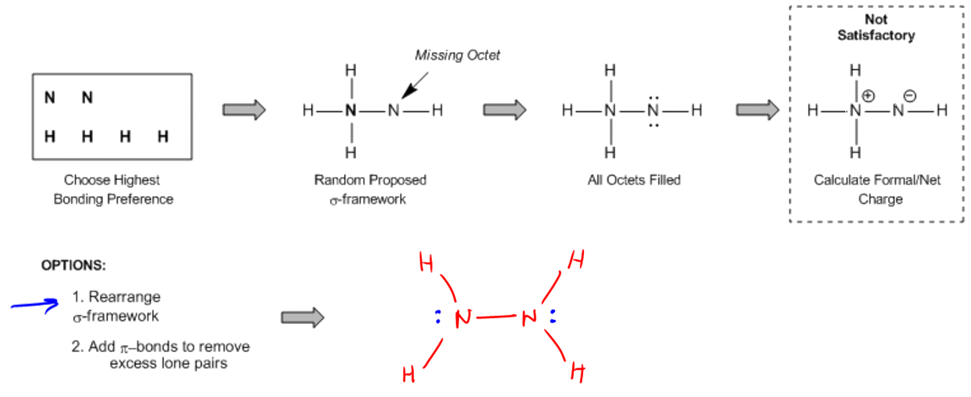In this example, we were left with a separation of charges (+ next to -), so we rearranged the σ-framework to see if we could remove them.

• This is a process of trial and error. Don’t be afraid to draw the wrong structure at first!

Practice: Draw the Lewis structure of the moleculePractice: Draw the Lewis structure of the molecule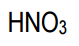Practice: Draw the Lewis structure of the molecule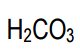Like the new method? Prefer the old one instead? Feel free to use whichever method you like more. As long as you get the right answer on the exam, that’s all I care about.